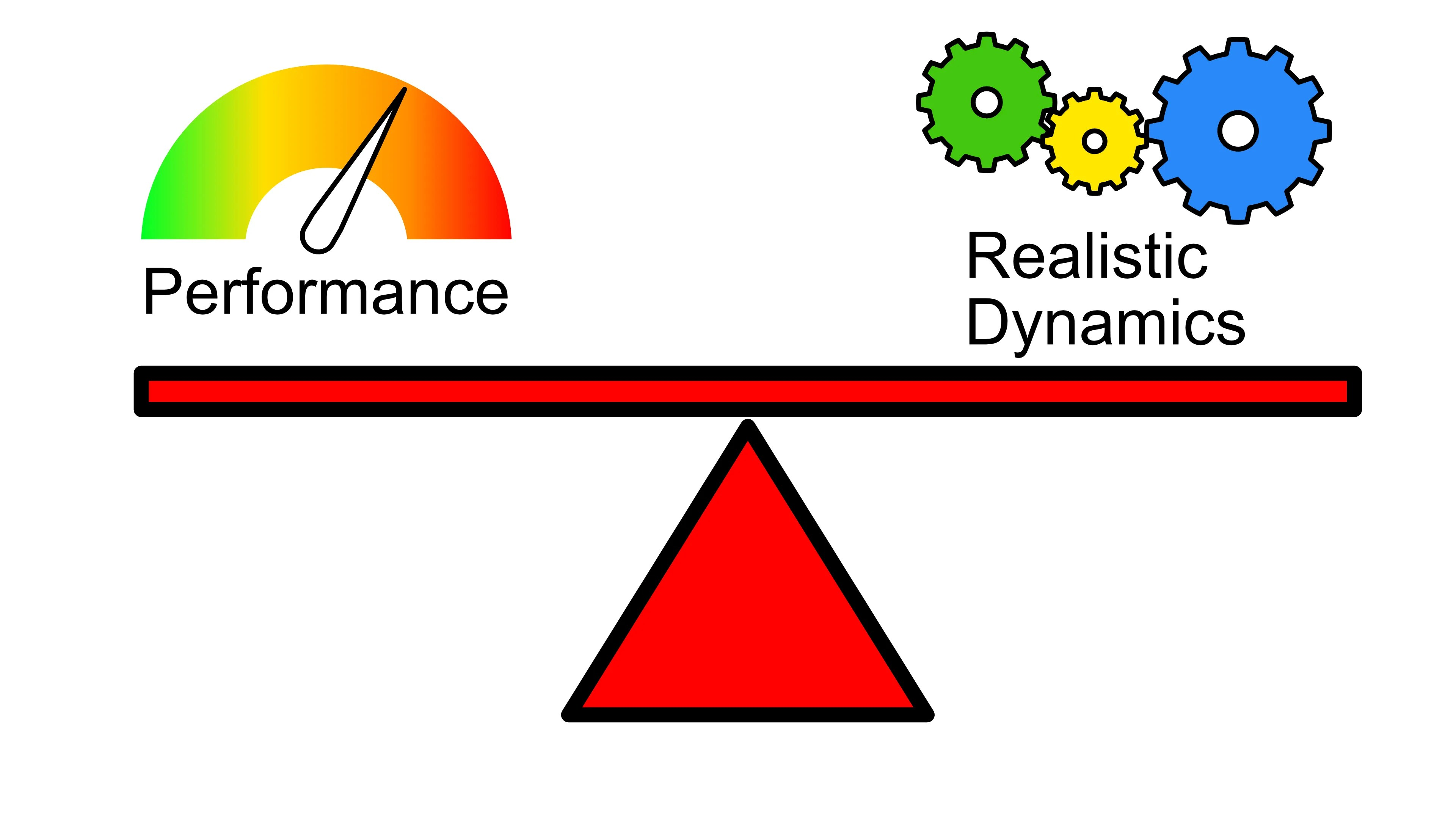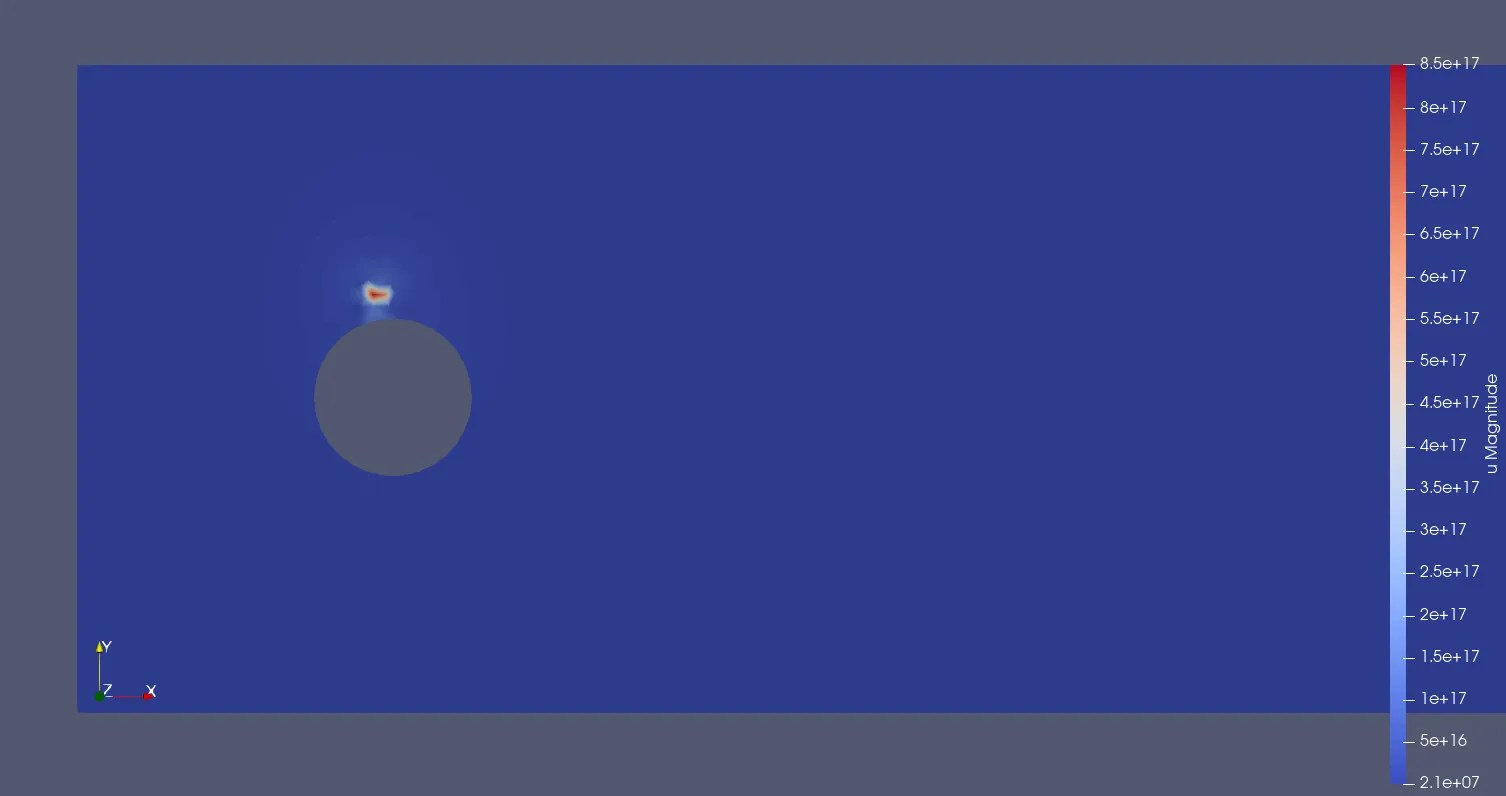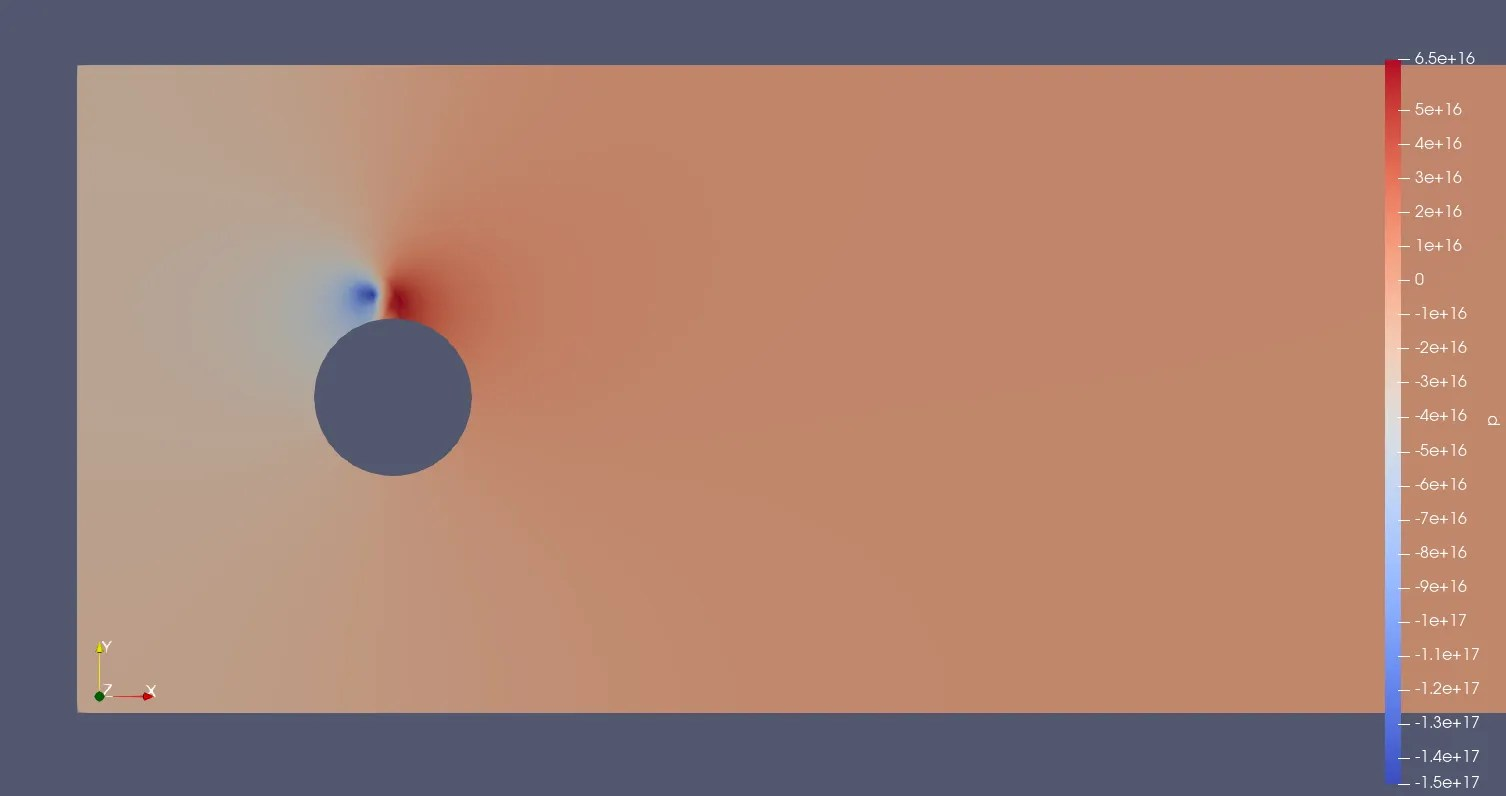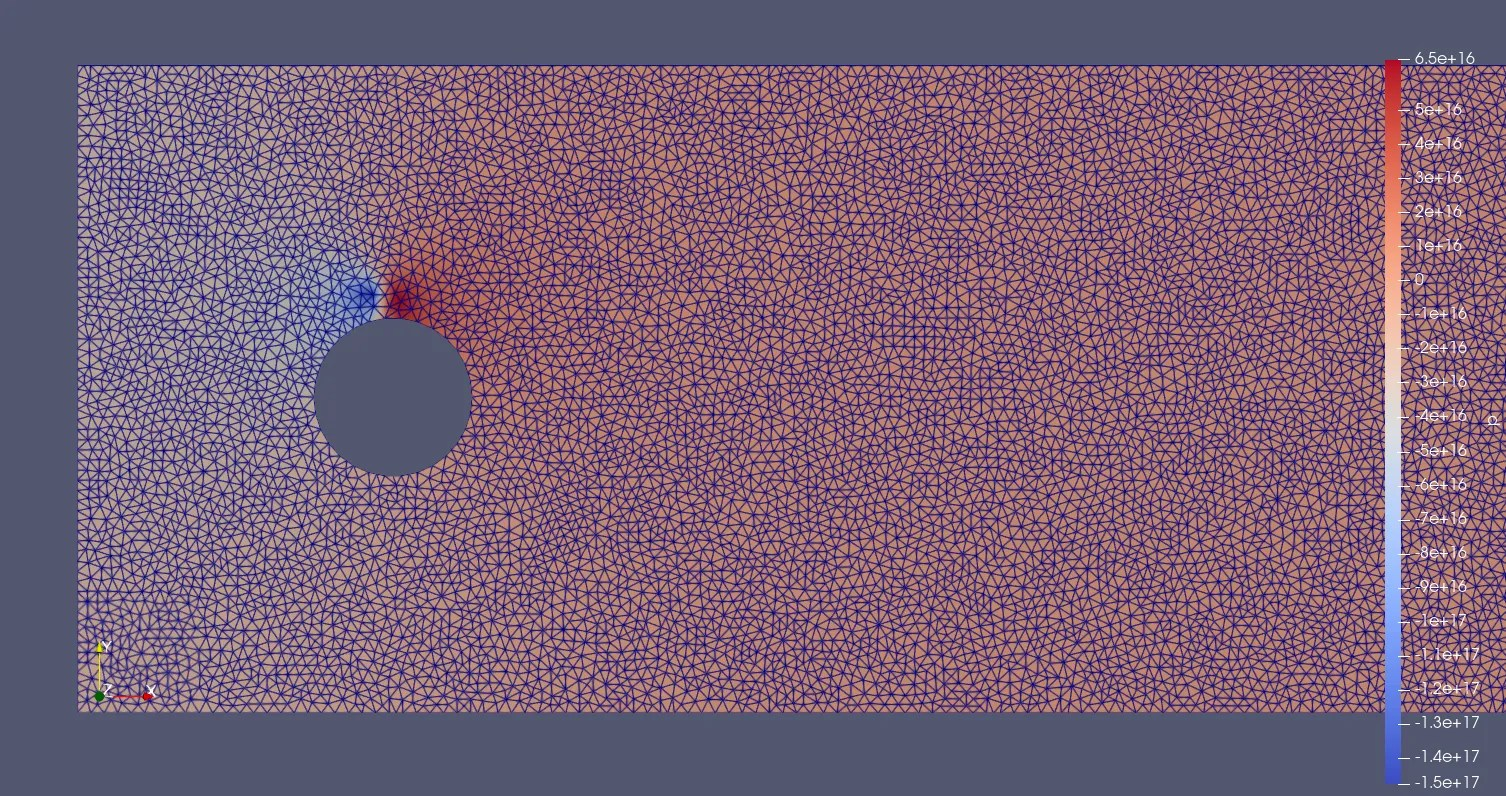# Why Is The Time-Step So Important?Figure 1: The balance of computational performance vs realistic dynamics for solving partial differential equations (PDEs). The time-step is one factor where increasing the time-step, increases the performance (the time required to solve the solution) but is at the cost of realistic dynamic modeling.

The time-step is fundamental for solving computational fluid dynamic problems (CFD) for unsteady, time-dependent problems. A balance between computational performance and realistic dynamics is often dependent on the time-step. It should be noted that all simulations make assumptions that are impacted by time-step and other factors such as the solver and mesh cell density. The time-step depends on a variety of factors from mesh cell size, velocity magnitude, solvers and iterations used. Additionally, the time-step may be limited due to the computational resources available with a lack of computational processing unit (CPU) power or lack of memory (RAM) for mesh modelling.

Typically, a large time-step can lead to unstable assumptions and solutions at each step of the solution. The assumptions do not model the flow dynamics correctly and may lead to exponential maximum velocity before the solution fails due to pressure and velocity overload, figure 2.

•Figure 1(a): Unstable Navier-Stoke solution for flow around the cylinder where flow becomes exponential between two mesh cells. The flow has reached around 1×10¹⁷ which is unrealistic. This exponential solution occurs when the time-step is too large and vectors pass across two mesh cell elements leading to unrealistic assumptions.
•Figure 1(b): Unstable Navier-Stoke solution for flow around the cylinder which becomes exponential between two mesh cells. Here mesh cells are shown with the surface elements filter. The flow has reached around 1×10¹⁷ which is unrealistic. This exponential solution occurs when the time-step is too large and vectors pass across two mesh cell elements leading to unrealistic assumptions.
•Figure 1(c): Unstable Navier-Stoke solution for flow around the cylinder where flow becomes exponential between two mesh cells. The pressure reached around 1×10¹⁶ which is unrealistic. This exponential solution occurs when the time-step is too large and the pressure gradient accounts for the velocity crossing two mesh cell elements leading to unrealistic assumptions.
•Figure 1(d): Unstable Navier-Stoke solution for flow around the cylinder where flow becomes exponential between two mesh cells. Here mesh cells are shown with the surface elements filter. The pressure reached around 1×10¹⁶ which is unrealistic. This exponential solution occurs when the time-step is too large and the pressure gradient accounts for the velocity crossing two mesh cell elements leading to unrealistic assumptions.
•Figure 1(e): Unstable Navier-Stoke solution for flow around the cylinder where flow becomes exponential between two mesh cells. Here a glyph overlay is used to highlight the flow direction around the exponential flow. The flow has reached around 1×10¹⁷ which is unrealistic. This exponential solution occurs when the time-step is too large and vectors pass across two mesh cell elements leading to unrealistic assumptions.

One solution is to reduce the time-step to a small value that never impacts the simulation and will always solve the CFD problem. However, reducing the time-step is at the expense of computational power where shorter time steps will lead to longer simulation times. Also, a shorter time-step is relative to the CFD problem. For one problem, a time-step of 1 x 10-2 seconds is appropriate whilst another problem has a time-step of 1 x 10-5 seconds, several magnitudes smaller. A method is required to calculate the maximum time-step.

## Courant-Friedrichs-Lewy (CFL) ConditionEquation 1: The Courant-Friedrichs-Lewy (CFL) condition where C is the Courant number, a is the maximum velocity, ΔT is the time-step and Δx is the minimum distance between mesh cells1. The Courant number is a dimensionless measure of a solution being solved within a mesh cell.

A method for calculating the maximum time-step is the Courant-Friedrichs-Lewy (CFL) condition1, equation 1. The CFL condition uses a Courant number, C, calculated from the maximum velocity, a, time-step, ΔT, and the minimum distance between mesh cells, Δx . The Courant number is a dimensionless number which is a measure of a solution being solved within a mesh cell. Ideally, solutions are solved for each cell avoiding assumptions over multiple mesh cells which can lead to instabilities. The CFL condition is re-arranged to calculate the maximum time-step. A Courant number of 1 will be the maximum theoretical time-step for simulating a stable solution as it is the smallest distance between mesh cells. A Courant number of 0.5 is recommended for an initial guess for a stable solution where it is smaller than the smallest mesh cell-size distance. This should ensure velocity vectors do not exceed several mesh cells and lead to large assumptions and an unstable simulation.

## Software

BERNAISE, Binary ElectRohydrodyNAmIc SolvEr, is an open-source, free CFD solver created in FEniCS – a solver for partial differential equations (PDEs). BERNAISE is a flexible high-level solver of electrohydrodynamic flows in complex geometries. It is written in Python and built on the FEniCS project, which in turn effectively interfaces to optimized linear algebra backends such as PETSc. The solver is described in Asger Bolet’s, Gaute Linga’s and Joachim Mathiesen’s paper.

This particular version of BERNAISE has been modified by myself to use .XDMF computer aided design (CAD) meshes. Additionally, I have added the CFL functionality in the IO scripts, get_dt_CFL(mesh, mu), which can be called in any of the BERNAISE problems. The function requires the mesh and the maximum velocity of the problem which is defined as the inlet flow.

## Step By Step

1. A CAD file can be used as well as a mesh generation by FEniCS / Dolfin. In this example, mesh is generated with FEniCS Dolfin. Documentation can be found here for FEniCS / Dolfin mesh generation. A full description of 2D and 3D CAD conversion is available on the BERNAISE wiki.
2. Assign parameters in the problem python file. In this case, the problem has been called flow_around_cylinder_scaled_CFL and the problem specific parameters are defined in the problem() function. More example problems can be found in the problems folder. The unsteady solver basic_IPCS_Adj is used with the get_dt_CFL to produce a CFL time-step.
1. The problem needs additional function from the common.io with get_dt_CFL(mesh, u) which required mesh and inflow velocity. This is added to line 5, from common.io import get_dt_CFL.
2. Using the get_dt_CFL changes the T to number of steps. The dt can be set as 0 as it will be automatically calculated from the mesh.
3. At line 132, the following code needs to be added which retrieves the mesh and inlet velocity passing to the get_dt_CFL function which returns the time step. The Courant number is automatically set to 0.5. Note: Use the top mesh function if using a FEniCS generated mesh or the second mesh function if using XDMF mesh within FEniCS.
``````# If using FEniCS generated mesh which we are here
mesh1 = mesh(parameters["H"], parameters["L"], parameters["x0"], parameters["y0"], parameters["R"], parameters["res"])

# If using an XDMF mesh within FEniCS
# Retrieve inlet dimensions (min/length) from mesh
[mesh1, parameters1] = mesh(parameters["mesh_file"],
parameters["subdomains_file"], parameters["XYZ"],
parameters["boundaries_Facet"], "inlet", parameters["scale_factor"], False)

# Use CFL (Courant-Friedrichs-Lewy) to calculate time step
dt = get_dt_CFL(mesh1, parameters["inlet_velocity"])
parameters["dt"] = dt * 1e-2
parameters["T"] = parameters["T"]*parameters["dt"]``````
1. Run the problem from the command prompt`python sauce.py problem=flow_around_cylinder_scaled`
2. View the exported .XDMF results in ParaView.

## Reviewing The Result

The CFL implementation in BERNAISE has been used in the curved micro-channel problem for part 1 of inertia microfluidics as it provided an initial starting point for iterating. However, studying the curved micro-channel problem (line 182), I used an additional scaling factor of 1 x 10-2 as larger time-steps lead to instabilities similar to flow around the cylinder, figure 1.

The instabilities were likely due to the calculation of the minimum distance between mesh elements. The minimum distance is calculated by the minimum cell size in BERNAISE. Also, additional dynamics may require a smaller time-step to model or the parameters are unrealistic for the CFD model. In the curved micro-channel case, reducing the time-step helped solve the problem and the parameters were realistic based upon the literature. One final factor can be the solver and iterative solving method.

The solver and iterative solving method was briefly discussed in Understanding the Navier-Stokes Solvers. Essentially different solving methods have iterative solving methods between time-steps. For example, the Chorin method has 3 steps where each iterate upon the previous solution making assumptions. The time-step can make the assumptions larger, which may require further iterations or lead to the Navier-Stokes solution failing outright.

## Conclusion

Setting the time-step is fundamental for solving CFD problems. Using the CFL condition, an educated estimation of the time-step can be used as the solution or a starting point to being iterating smaller time-steps off. The implementation is not perfect in BERNAISE likely due to the method of obtaining the smallest distance between mesh cells. Ultimately, the CFL condition presented aids in producing a rough upper time-step limit for CFD solutions.

## References

1. Courant, R., Friedrichs, K., & Lewy, H. (1928). Über die partiellen Differenzengleichungen der mathematischen Physik. Mathematische Annalen, 100(1), 32–74. https://doi.org/10.1007/BF01448839